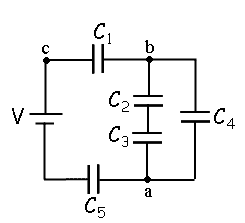# Charge on a capacitor in a series

## Homework Statement[/B]
A circuit is constructed with five capacitors and a battery as shown. The values for the capacitors are: C1 = C5 = 3.9 μF, C2 = 3.9 μF, C3 = 5.7 μF, and C4 = 4.2 μF. The battery voltage is V = 12 V.

What is Q5, the charge on capacitor C5?

C=Q/V

## The Attempt at a Solution

Okay, I thought that voltage across series capacitors was all the same across each one. Treating the parallel system as one capacitor of equivalent capacitance, I thought you could just divide the voltage by 3, and then multiply by C5 to get the charge on C5. I tried this and the answer is wrong. I'm really confused.

ehild
Homework Helper

## Homework Statement[/B]
A circuit is constructed with five capacitors and a battery as shown. The values for the capacitors are: C1 = C5 = 3.9 μF, C2 = 3.9 μF, C3 = 5.7 μF, and C4 = 4.2 μF. The battery voltage is V = 12 V.

What is Q5, the charge on capacitor C5?

C=Q/V

## The Attempt at a Solution

Okay, I thought that voltage across series capacitors was all the same across each one. Treating the parallel system as one capacitor of equivalent capacitance, I thought you could just divide the voltage by 3, and then multiply by C5 to get the charge on C5. I tried this and the answer is wrong. I'm really confused.

The voltage across capacitors in series is not the same, unless the capacitances are equal. What is the resultant of C2, C3 and C4? Is it equal to 3.9 μF?
I think you mix charge with voltage. The charge is same on series capacitors .